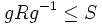# Fusion system induced by a finite group on its p-Sylow subgroup

Suppose$G$ is a finite group,$p$ is a prime number, and$P$ is a$p$-Sylow subgroup. The fusion system on$P$ induced by$G$ is defined as follows: for every element$g \in G$, and subgroups$R,S \le P$ such that$gRg^{-1} \le S$, there is a morphism$\varphi:R \to S$ given by$\varphi(r) = grg^{-1}$.
This fusion system is often written as$\mathcal{F}_P(G)$. The special case where$G = P$ gives rise to what we call the inner fusion system.